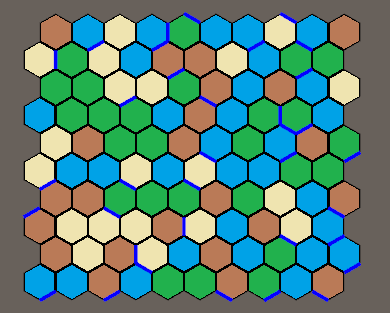HexMapLibrary
Example 1: Mouse markers and simple map manipulation

In this example we are going to create a HexMap with a rectangular shape and will assign a random value to each of those tiles which we will visualise by using different colors. We then will add the HexMouse component to get the position of the mouse and display the tile the cursor is on as well as the closest tile and edge. When clicking a tile it will change its value.

As in the previous example we will add the using statements for our library and position the camera correctly so these things are not covered again here.

This time we will need a few more prefabs & variables :

[SerializeField] private Vector2Int mapSize = new Vector2Int(13, 13);
[SerializeField] private GameObject tilePrefab = null; // the prefab we use for each Tile -> use TilePrefab.prefab
[SerializeField] private GameObject edgePrefab = null; // the prefab we use for each Edge -> use EdgePrefab.prefab
[SerializeField] private GameObject tileMarker = null; // a GameObject we use to show the current mouse position -> put an instance of TileMarker.prefab in the scene and reference it in the inspector
[SerializeField] private GameObject edgeMarker = null; // a GameObject we use to we show the closest edge to the current mouse position -> put an instance of EdgeMarker.prefab in the scene and reference it in the inspector
[SerializeField] private GameObject cornerMarker = null; // a GameObject we use to show the closest corner to the current mouse position -> put an instance of CornerMarker.prefab in the scene and reference it in the inspector
[SerializeField] private List<Material> materials = null; // the materials we want to assign to the tiles for visualisation purposes -> set size to 4 in inspector and add TileMat1 to TileMat4
private HexMap<int,bool> hexMap; // our map. For this example we create a map where an integer represents the data of each tile and a bool the data of each edge
private HexMouse hexMouse = null; // the HexMouse component we add to keep track of the mouse position
private GameObject[] tileObjects; // this will contain all the GameObjects for visualisation purposes, their array index corresponds with the index of our Tiles

Now it is time to create our map:

void Start ()
{
hexMap = new HexMap<int, bool>(HexMapBuilder.CreateRectangularShapedMap(mapSize), null); //creates a HexMap using one of the pre-defined shapes in the static MapBuilder Class
hexMouse = gameObject.AddComponent<HexMouse>(); //we attach the HexMouse script to the same gameObject this script is attached to, could also attach it anywhere else
hexMouse.Init(hexMap); //initializes the HexMouse
tileObjects = new GameObject[hexMap.TilesByPosition.Count]; //creates an array with the size equal to the number on tiles of the map
foreach (var tile in hexMap.Tiles) //loops through all the tiles, assigns them a random value and instantiates and positions a gameObject for each of them.
{
tile.Data = (Random.Range(0, 4));
GameObject instance = GameObject.Instantiate(tilePrefab);
instance.GetComponent<Renderer>().material = materials[tile.Data];
instance.name = "MapTile_" + tile.Position;
instance.transform.position = tile.CartesianPosition;
tileObjects[tile.Index] = instance;
}
foreach (var edge in hexMap.Edges) //we randomly spawn a GameObject on some corners (could be representing walls or rivers or anything else)
{
int randomNumber = Random.Range(0, 100);
if (randomNumber > 89)
{
edge.Data = true;
GameObject instance = GameObject.Instantiate(edgePrefab);
instance.name = "MapEdge_" + edge.Position;
instance.transform.position = edge.CartesianPosition;
instance.transform.rotation = Quaternion.Euler(0, edge.EdgeAlignmentAngle, 0);
//as we don't change the edges during runtime we don't need to add the gameObjects of the edges to an array as we don't need to manipulate them later on
}
}
SetupCamera(); //put the camera related lines from previous script in that method
}

If you run the scene now, it should look similar to this:Finally we add our update method which will highlight the current tile and closest edge of our mouse position and when we leftclick we will change the value of the current tile

void Update ()
{
if (!hexMouse.CursorIsOnMap) return; // if we are not on the map we won't do anything so we can return
Vector3Int mouseTilePosition = hexMouse.TileCoord;
Vector3Int mouseEdgePosition = hexMouse.ClosestEdgeCoord;
Vector3Int mouseCornerPosition = hexMouse.ClosestCornerCoord;
//update the marker positions
tileMarker.transform.position = HexConverter.TileCoordToCartesianCoord(mouseTilePosition, 0.1f); //we put our tile marker on the tile our mouse is on
edgeMarker.transform.position = HexConverter.EdgeCoordToCartesianCoord(mouseEdgePosition); // we put our edge marker on the closest edge of our mouse position
edgeMarker.transform.rotation = Quaternion.Euler(0, hexMap.EdgesByPosition[mouseEdgePosition].EdgeAlignmentAngle, 0); //we set the rotation of the edge marker
cornerMarker.transform.position = HexConverter.CornerCoordToCartesianCoord(mouseCornerPosition);
if (Input.GetMouseButtonDown(0)) // change a tile when clicked on it
{
Tile<int> t = hexMap.TilesByPosition[mouseTilePosition]; //we select the tile our mouse is on
int curValue = t.Data; //we grab the current value of the tile
t.Data = ((curValue + 1) % 4); //we increment it and use modulo to keep it between 0 and 3
tileObjects[t.Index].GetComponent<Renderer>().material = materials[t.Data]; // we update the material of the GameObject representing the tile based on the new value
}
}

This concludes this example, now let's continue with the next [ADD LINK]